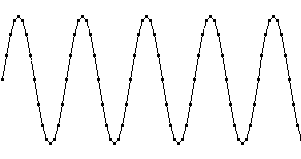Daqarta
Data AcQuisition And Real-Time Analysis
Scope - Spectrum - Spectrogram - Signal Generator
Software for WindowsThe following is from the Daqarta Help system:

# Sampled Data for the Discrete Fourier Transform

Unlike the continuous Fourier Transform just described, the Discrete Fourier Transform (DFT) uses digital methods that allow computer inplementation. In place of a continuous input signal we will need sampled data from an Analog-to-Digital Converter (ADC). This device takes samples of the input signal at regular intervals and outputs a digital number proportional to the instantaneous voltage.

Here is an example of a sampled sine wave. The solid black squares mark the actual data samples:The time between samples is called the sample period, and the rate of sampling is called (surprise!) the sample rate or sampling frequency. No information is available about what the input does between samples, so we must make sure that we sample often enough to adequately represent its behavior. In this example, the actual input sine is at 3 kHz, and the solid black squares mark the individual samples that result from sampling at 48000 samples per second. That sample rate is 16 times the signal frequency, so there are 16 samples per cycle. This allows a perfect reconstruction of the input from the data, as shown by the black curve.

Given only the sample values, the black curve could have simply been drawn "by eye" in a connect-the-dots fashion. In general, however, we must consider the sinusoidal nature of the signal. When there are few samples per cycle, a straight-line approximation would give a poor representation of the waveform. Nevertheless, an DFT analysis could provide the frequency, amplitude, and phase parameters needed to reproduce the input exactly.

What is the minimum sample rate that will accurately capture the details of an input signal? Or, given a certain sample rate, what limits are imposed on input signals if they are to be perfectly captured? The limits are determined by a phenomenon called aliasing.GO: